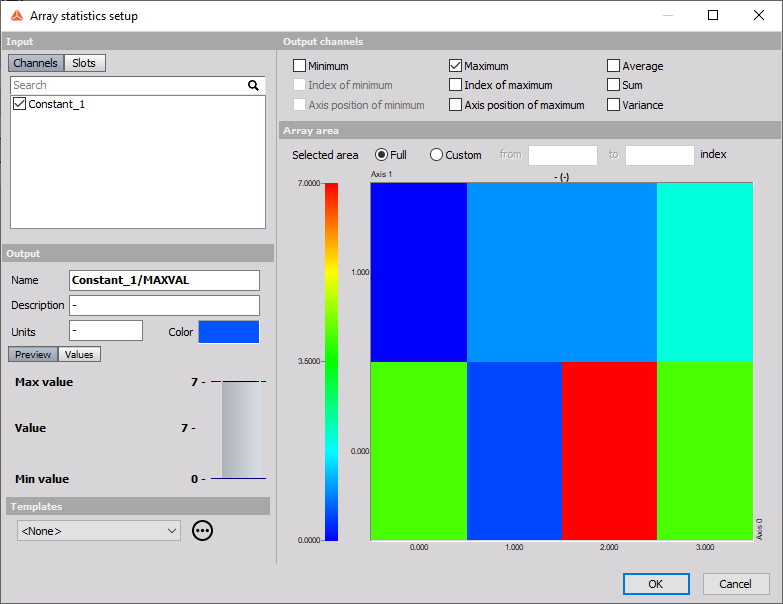# Array statistics

The array statistics can calculate the statistical value from the array.

There are several options which can be chosen:

• Minimum finds minimum value from the array. There are two output channels created: class and value. The class will describe which index of the array holds the parameter and the value will be the minimum value itself.
• Maximum finds maximum value from the array. There are two output channels created: class and value. The class will describe which index of the array holds the parameter and the value will be the maximum value itself.
• Average calculates the average value of all elements from the array.
• Sum calculates the sum of all elements from the array.
• Variance calculates the variance of all elements from the array.